JEE  >  Graphs for Physics

# Graphs for Physics - Notes | Study Physics For JEE - JEE

 Table of contentsCommon Graph Forms1 Crore+ students have signed up on EduRev. Have you?

Common Graph Forms

Working with graphs – interpreting, creating, and employing – is an essential skill in the sciences, and especially in physics where relationships need to be derived. As an introductory physics student you should be familiar with the typical forms of graphs that appear in physics. Below are a number of typical physical relationships exhibited graphically using standard X-Y coordinates (e.g., no logarithmic, power, trigonometric, or inverse plots, etc.). Study the forms of the graphs carefully, and be prepared to use the program Graphical Analysis to formulate relationships between variables by using appropriate curve-fitting strategies. Note that all non-linear forms of graphs can be made to appear linear by “linearizing” the data. Linearization consists of such things as plotting X versus Y2 or X versus 1/Y or Y versus log (X), etc. Note: While a 5th order polynomial might give you a better fit to the data, it might not represent the simplest model.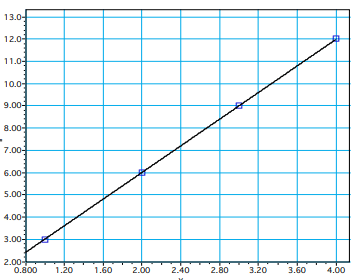Linear Relationship: What happens if you get a graph of data that looks like this? How does one relate the X variable to the Y variable? It’s simple, Y = A + BX where B is the slope of the line and A is the Y-intercept. This is characteristic of Newton’s second law of motion and of Charles’ law: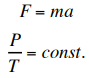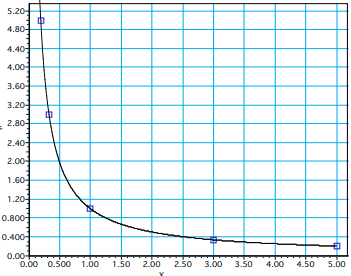Inverse Relationship: This might be a graph of the pressure and temperature for a changing volume constant temperature gas. How would you find this relationship short of using a computer package? The answer is to simplify the plot by manipulating the data. Plot the Y variable versus the inverse of the X variable. The graph becomes a straight line. The resulting formula will be Y = A/X or XY = A. This is typical of Boyle’s law:
PV = const.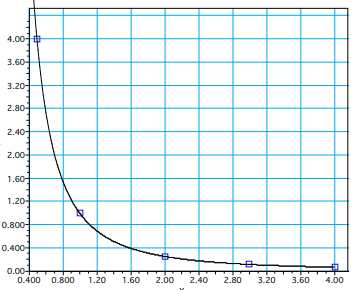Inverse-Square Relationship: Of the form Y = A/X2. Characteristic of Newton’s law of  universal gravitation, and the electrostatic force law: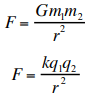In the latter two examples above there are only subtle differences in form. Many common graph

forms in physics appear quite similar. Only be looking at the “RMSE” (root mean square error

provided in Graphical Analysis) can one conclude whether one fit is better than another. The better fit is the one with the smaller RMSE.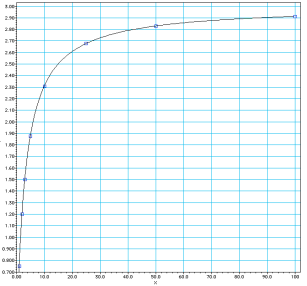Double-inverse Relationship: Of the form 1/Y = 1/X + 1/A. Most readily identified by the presence of an asymptotic boundary (y = A) within the graph. This form is characteristic of the thin lens and parallel resistance formulas.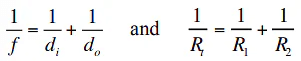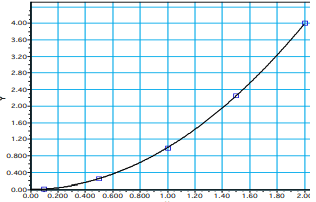Power Relationship: Top opening parabola. Of the form Y = AX2. Typical of the distance-time relationship: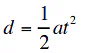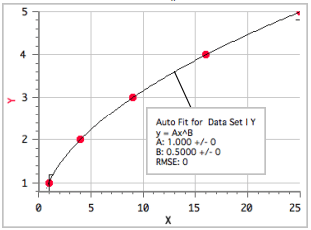Power Relationship:  Side opening parabola. Of the formY2 = A1X or Y=A2X1/2. Typical of the simple pendulum relationship: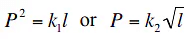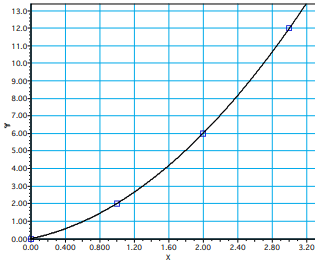Polynomial of the Second Degree: Of the form Y =AX + BX2. Typical of the kinematics equation: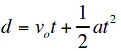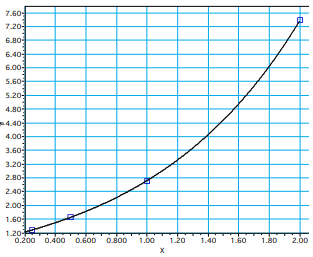Exponential Relationship: Of the form Y = A * exp(BX). Characteristic of exponential growth or decay. Graph to left is exponential growth. The graph of exponential decay would look not unlike that of the inverse relationship. Characteristic of radioactive decay.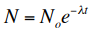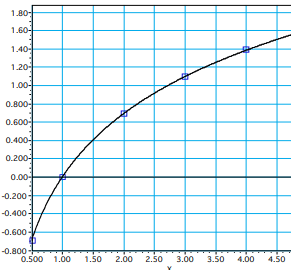Natural Log (Ln) Relationship: Of the form Y = A ln(BX). Characteristic of entropy change during a free expansion: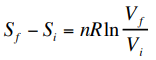The document Graphs for Physics - Notes | Study Physics For JEE - JEE is a part of the JEE Course Physics For JEE.
All you need of JEE at this link: JEE

## Physics For JEE

257 videos|633 docs|256 tests
 Use Code STAYHOME200 and get INR 200 additional OFF

## Physics For JEE

257 videos|633 docs|256 tests

Track your progress, build streaks, highlight & save important lessons and more!

,

,

,

,

,

,

,

,

,

,

,

,

,

,

,

,

,

,

,

,

,

;